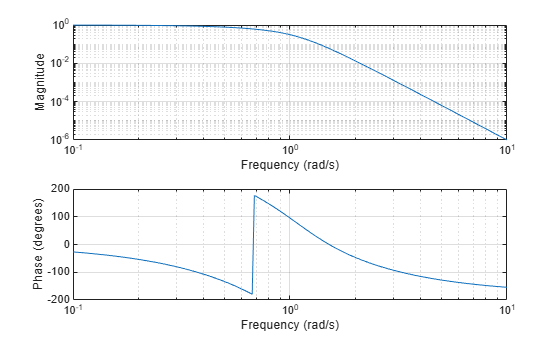# besselap

Bessel analog lowpass filter prototype

## Syntax

```[z,p,k] = besselap(n) ```

## Description

`[z,p,k] = besselap(n)` returns the poles and gain of an order-`n` Bessel analog lowpass filter prototype. `n` must be less than or equal to 25. The function returns the poles in the length `n` column vector `p` and the gain in scalar `k`. `z` is an empty matrix because there are no zeros. The transfer function is

`$H\left(s\right)=\frac{k}{\left(s-p\left(1\right)\right)\left(s-p\left(2\right)\right)\cdots \left(s-p\left(n\right)\right)}$`

`besselap` normalizes the poles and gain so that at low frequency and high frequency the Bessel prototype is asymptotically equivalent to the Butterworth prototype of the same order . The magnitude of the filter is less than $1/\sqrt{2}$ at the unity cutoff frequency Ωc = 1.

Analog Bessel filters are characterized by a group delay that is maximally flat at zero frequency and almost constant throughout the passband. The group delay at zero frequency is

`${\left(\frac{\left(2n\right)!}{{2}^{n}n!}\right)}^{1/n}$`

## Examples

collapse all

Design a 6th-order Bessel analog lowpass filter. Display its magnitude and phase responses.

```[z,p,k] = besselap(6); % Lowpass filter prototype [num,den] = zp2tf(z,p,k); % Convert to transfer function form freqs(num,den) % Frequency response of analog filter```## Algorithms

`besselap` finds the filter roots from a lookup table constructed using Symbolic Math Toolbox™ software.

## References

 Rabiner, L. R., and B. Gold. Theory and Application of Digital Signal Processing. Englewood Cliffs, NJ: Prentice-Hall, 1975, pp. 228–230.